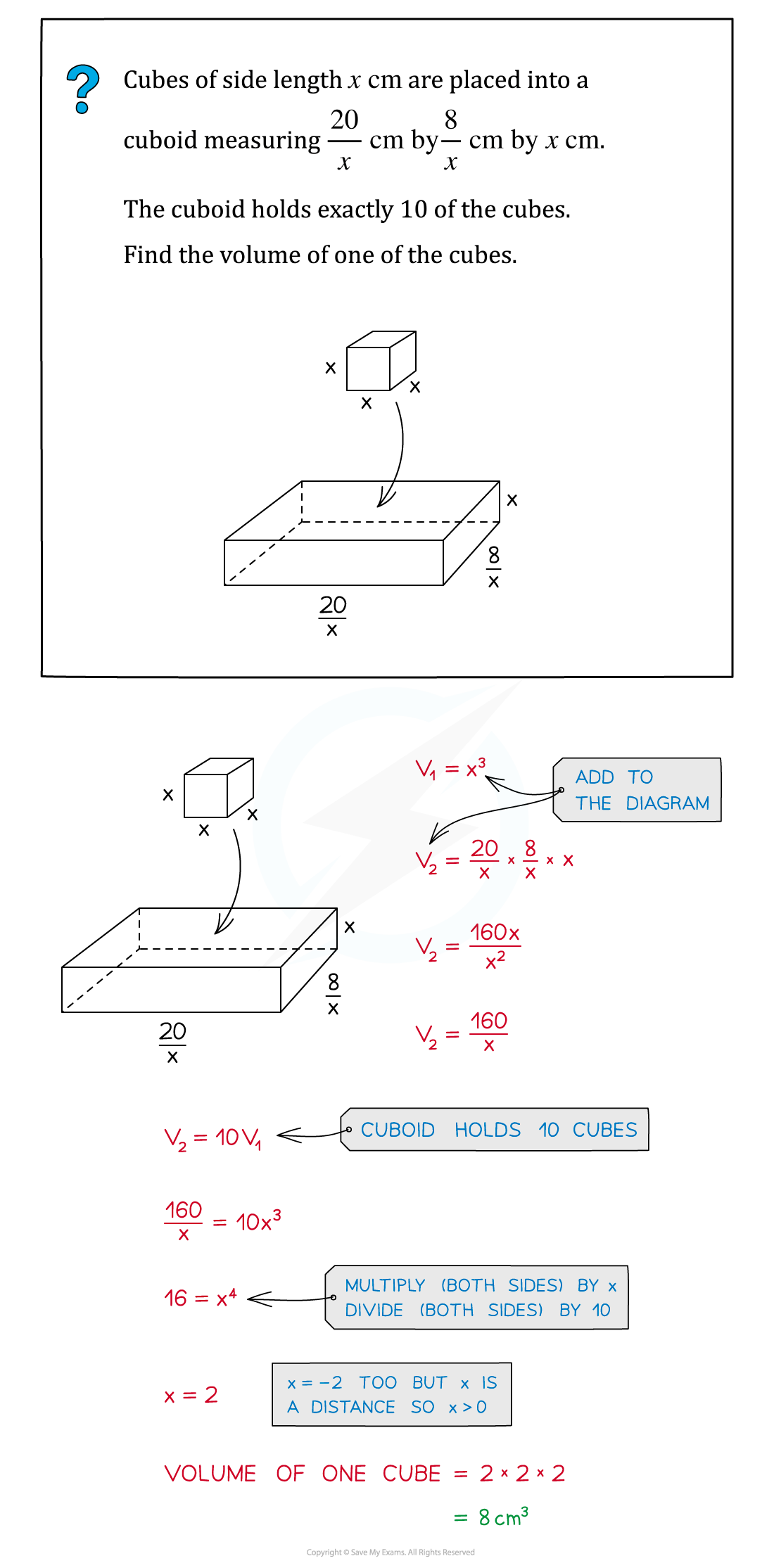# Edexcel IGCSE Maths 复习笔记 2.10.1 Equations & Problem Solving

Edexcel IGCSE Maths 复习笔记 2.10.1 Equations & Problem Solving

#### What is problem solving?

• Problem solving in mathematics involves using several stages, across a variety of topics, to answer a question
• In this set of notes all the problems will involve equations
• These could be linear equations, quadratic equations or simultaneous equations and other, relatively straightforward equations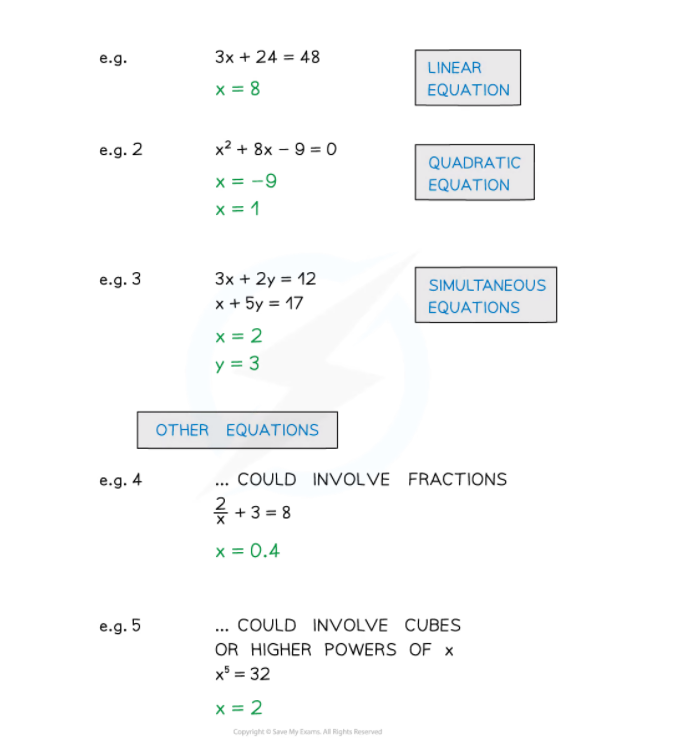• You may notice there are not many subheadings in these notes
• That is deliberate so the examples are not labelled or dealt with in an order
• This is the nature of problem solving questions!
• You never know exactly what’s coming ... !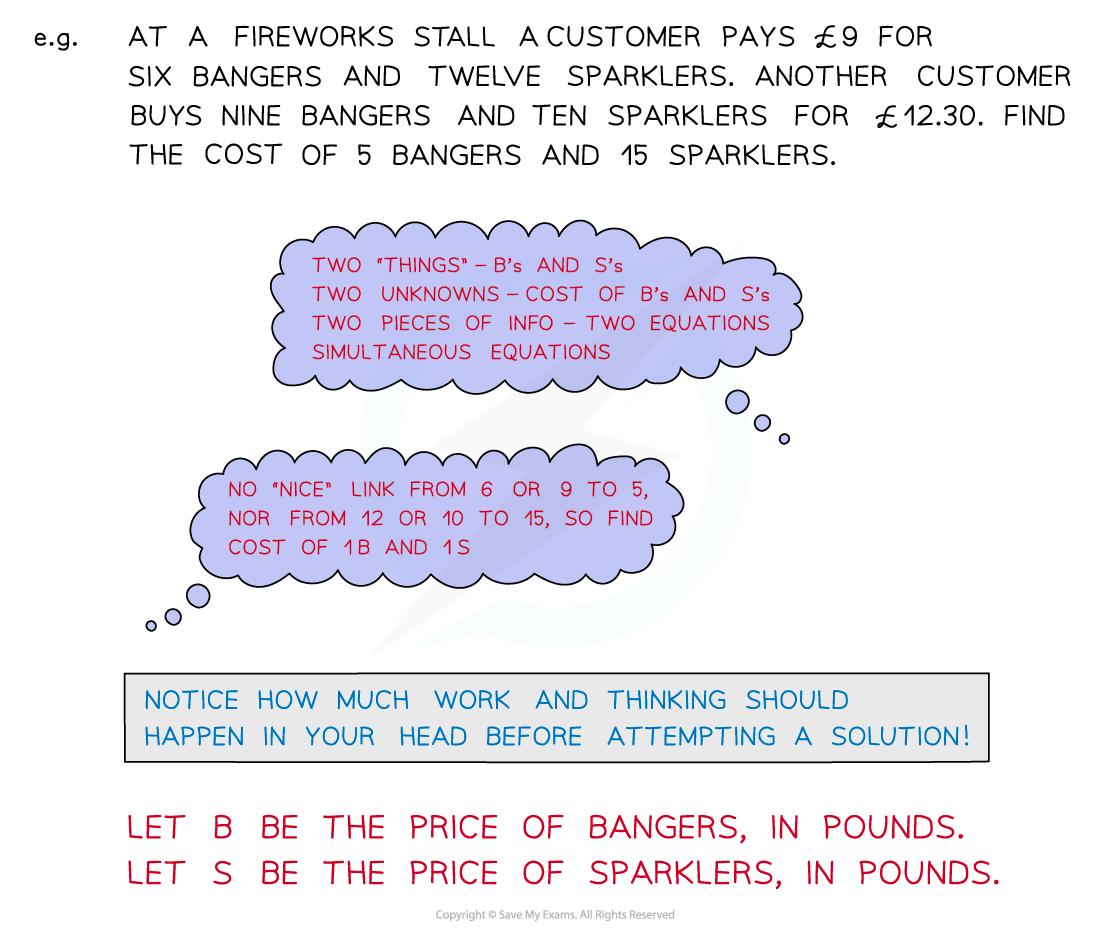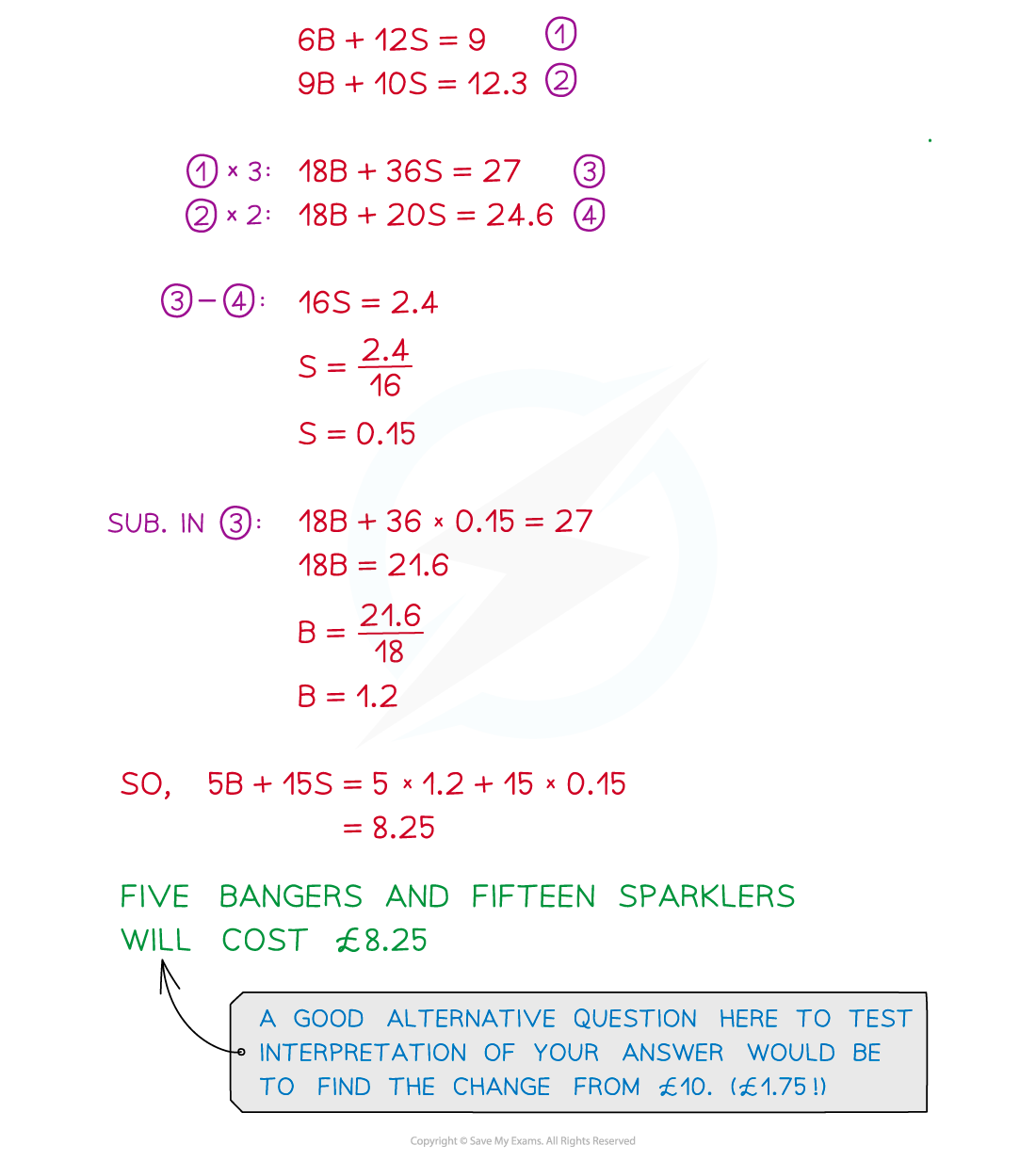• In an ordinary mathematics question you would be given an equation to solve
• In a problem solving question you would have to generate the equation ...
• ... using information from the question
• ... using your knowledge of standard mathematical results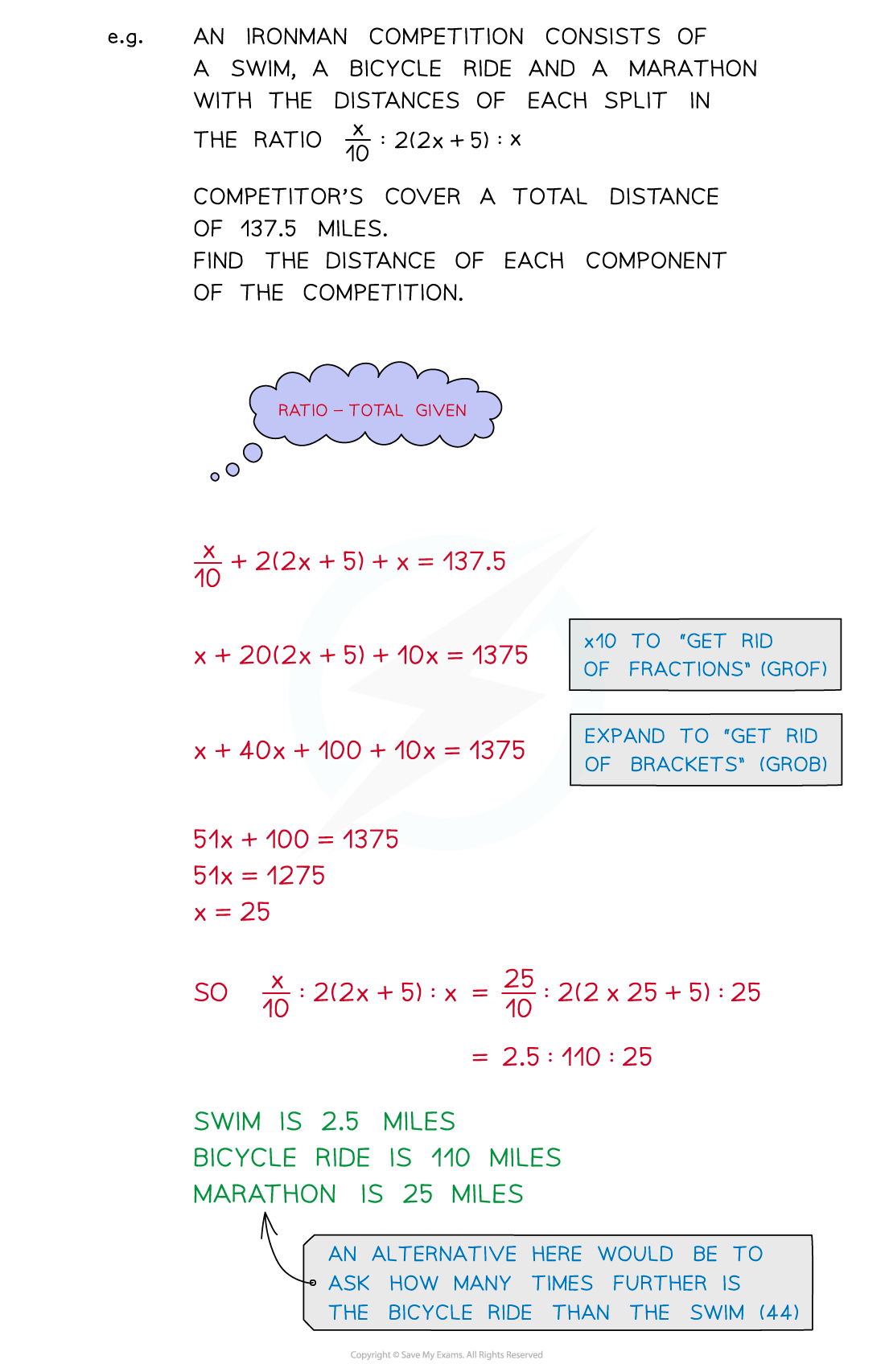• A key feature of problem solving questions is to interpret the answer in context
• An answer on a calculator may be 2

• quadratic equation can have two solutions
• Only one may be valid if only positive values are relevant (eg distance)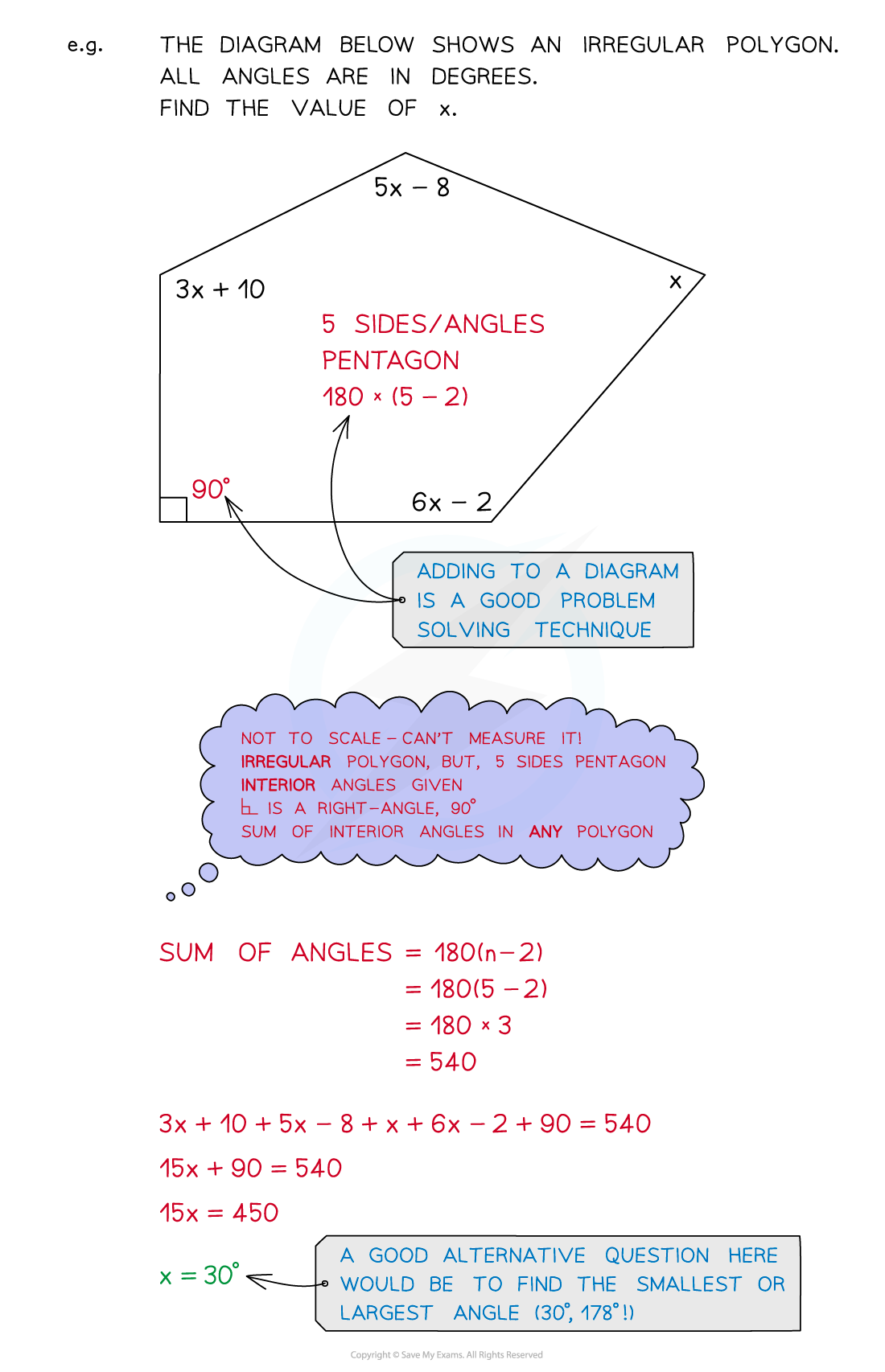• In problem solving questions you are typically given less information about the type of maths involved
• It is impossible to list every type of problem solving question you could see
• There are endless contexts questions can be set in
• There is no one-fits-all step-by-step method to solving problems

• Practiceexperience and familiarity are the keys to solving problems successfully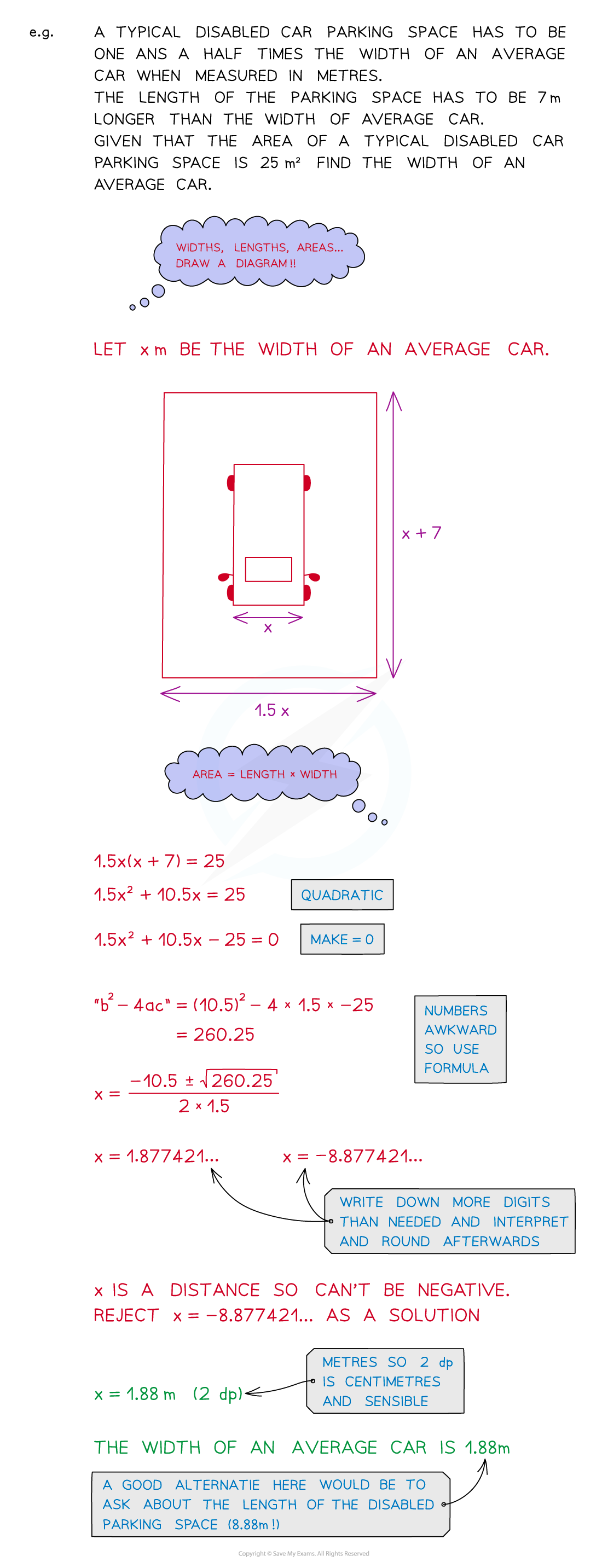• Do not necessarily expect whole number (integer) or “nice” solutions
• Especially where a calculator is allowed

• Rounding appropriately may be one of the skills being tested
• eg Rounding a value in cm only needs to be to one decimal place;so it indicates mm

#### Exam Tip

Do not start by focusing on what the question has asked you to find, but on what maths you can do.If your attempt turns out to be unhelpful, that’s fine, you may still pick up some marks.If your attempt is relevant it could nudge you towards the full solution – and full marks!Add information to a diagram as you work through a problem. If there is no diagram, try sketching one.

#### Worked Example